# Extensible Pendulum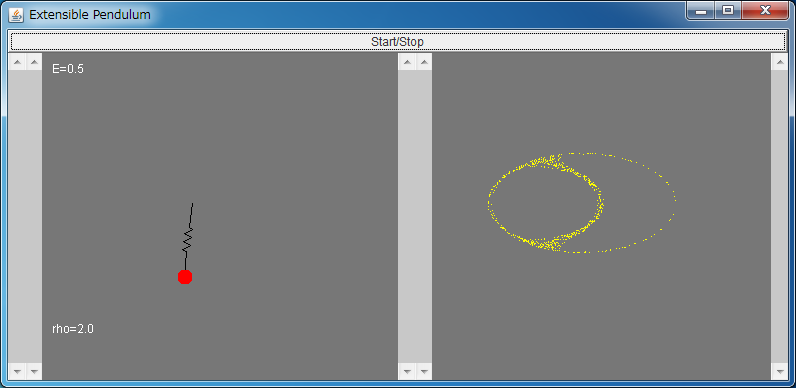If the above application does not start, please install OpenJDK from adoptium.net.

[for E=1.036, rho=1.92]
• Chaos (1)
• Torus (1 / 2)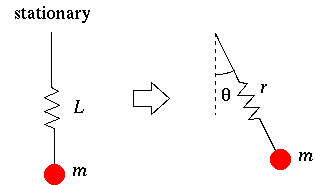Let's consider the extensible pendulum as shown in the above figure.

For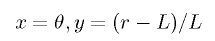, the following differential equation holds.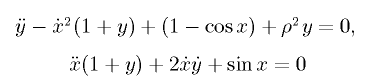where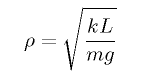and k is the stiffness.

This simulator numerically integrates the above differential equation and visualizes its dynamics.
Similarly to the double pendulum simulator, chaos can be observed for some initial conditions and parameters.